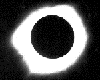PHYSICS IN AUSTRALIA TO 1945

Inquiries and corrections to R.W. Home (home@unimelb.edu.au)
WWW edition prepared by Tim Sherrat and Victoria Young for Bright Sparcs, June 1995

## CARSLAW, Horatio Scott

Born Helensburgh, Scotland, 12 February 1870; died Burradoo, N.S.W., 11 November 1954. Education University: Glasgow (M.A. 1891), Cambridge University (4th wrangler 1894, Sc.D. 1908) and University: G#eottingen (1896-97). Fellow of Emmanuel College Cambridge, 1899-1905. Assistant lecturer in mathematics University of Glasgow 1896-1903. Professor in mathematics at Sydney University 1903-35.
1. The steady motion of a spherical vortex. Edinburgh Mathematical Society. Proceedings., 15 (1896-97), 76-80.
2. The fluted vibrations of a circular vortex ring with a hollow core. London Mathematical Society. Proceedings., 28 (1897), 97-119.
3. Note on the transformations of the equations of hydrodynamics. Edinburgh Mathematical Society. Proceedings., 16 (1897-98), 36-38.
4. Some muiltiform solutions of the partial differential equations of physical mathematics and their applications. London Mathematical Society. Proceedings., 30 (1899), 121-163.
5. The use of multiple space in applied mathematics. British Association for the Advancement of Science. Report of the Annual Meeting., (Bradford, 1900), 644-646.
6. Oblique incidence of a train of plane waves on a semi-infinite plane. Edinburgh Mathematical Society. Proceedings., 19 (1900-01), 71-77.
7. A problem in conduction of heat. Philosophical Magazine, S.6, 4 (1902), 162-165.
8. The use of contour integration in the problem of diffraction by a wedge of any angle. Philosophical Magazine, S.6, 5 (1903), 374-379.
9. The use of Green's functions in the mathematical theory of the conduction of heat. Edinburgh Mathematical Society. Proceedings., 21 (1902-03), 40-64.
10. The scattering of waves by a cone. Philosophical Magazine, S.6, 20 (1910), 690-691.
11. The Green's function for a wedge of any angle, and other problems in the conduction of heat. London Mathematical Society. Proceedings., S.2, 8 (1910), 365-374.
12. A problem in the linear flow of heat, discussed from the point of view of the theory of integral equations. Edinburgh Mathematical Society. Proceedings., 30 (1911-12), 97-106.
13. Integral equations and the determination of Green's functions in the theory of potential. Edinburgh Mathematical Society. Proceedings., 31 (1912-13), 71-89.
14. Functions of positive type and their application to the determination of Green's functions. Messenger of Mathematics, 42 (1912-13), 135-140.
15. The relation between pure and applied mathematics. Australasian Association for the Advancement of Science. Report of Meeting., 14 (Melbourne, 1913), 6-18.
16. The scattering of sound waves by a cone. Mathematische Annalen, 75 (1914), 133-147.
17. The Green's function for the equation nabla²u + k²u = 0. London Mathematical Society. Proceedings., S.2, 13 (1914), 236-257.
18. The Green's function for the equation nabla²u + k²u = 0. Second paper. London Mathematical Society. Proceedings., S.2, 16 (1917), 84-93.
19. Problems in conduction of heat. Philosophical Magazine, S.6, 38 (1919), 200.
20. Diffraction of waves by a wedge of any angle. London Mathematical Society. Proceedings., S.2, 18 (1920), 291-306.
21. Bromwich's method of solving problems in the conduction of heat. Philosophical Magazine, S.6, 39 (1920), 603-611.
22. The cooling of a solid sphere with a concentric core of a different material. Cambridge Philosophical Society. Proceedings., 20 (1921), 399-410.
23. Operational methods in mathematical physics. Mathematical Gazette, 14 (1928), 216-225.
24. Operational methods in mathematical physics. Mathematical Gazette, 22 (1938), 264-280.
25. (With J.C. Jaeger) Some problems in the mathematical theory of the conduction of heat. Philosophical Magazine, S.7, 26 (1938), 473-495.
26. (With J.C. Jaeger) On Green's functions in the theory of heat conduction. American Mathematical Society. Bulletin., 45 (1939), 407-413.
27. (With J.C. Jaeger) A problem in conduction of heat. Cambridge Philosophical Society. Proceedings., 35 (1939), 394-404.
28. (With J.C. Jaeger) The determination of Green's function for the equation of conduction of heat in cylindrical coordinates by the Laplace transformation. London Mathematical Society. Journal., 15 (1940), 273-281.
29. (With J.C. Jaeger) Some two-dimensional problems in conduction of heat with circular symmetry. London Mathematical Society. Proceedings., S.2, 46 (1940), 361-388.
30. A simple application of the Laplace transformation. Philosophical Magazine, S.7, 30 (1940), 414-417.
31. (With J.C. Jaeger) The determination of Green's function for line sources for the equation of conduction of heat in cylindrical coordinates by the Laplace transformation. Philosophical Magazine, S.7, 31 (1941), 204-208.
32. Introduction to the theory of Fourier's series and integrals and the mathematical theory of the conduction of heat. London: Macmillan, 1906. xvii + 434pp. 2nd, revised edition in two vols., Introduction to the theory of Fourier's series and integrals (London: Macmillan, 1921), xii + 323pp., and Introduction to the mathematical theory of the conduction of heat in solids (London: Macmillan, 1921), xii + 268pp.; Japanese translation by Takemae Genzo: Fourier kyûsû oyobi sekibun ron (Tokyo: Uchida-Rôkaku-Ho, 1927), xii + 482 pp., and Netsu dendô ron (Tokyo: Uchida-Rôkaku-Ho, 1928), xv + 405 pp.
33. (With J.C. Jaeger) Operational methods in applied mathematics. Oxford: Clarendon Press, 1941. xvi + 264p.

[ Contents | Previous entry | Next entry | Bright SPARCS | ASAPWeb ]